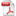# The Planck-Shannon Classifier Algorithm

There are many natural and human phenomena in the physical, biomedical, and social sciences that generate long-tailed asymmetric histograms (LTAH) that fit the Planckian Distribution Equation (PDE).  It has been postulated that the Planckian process (defined as the physicochemical, socioeconomic, or cosmological processes that generate LTAH’s fitting PDE) involves a selection mechanism that can identify as special a subset of events out of all possible randomly organized events .Figure 1. Some examples of long-tailed asymmetric histograms (LTAH's) that fit PDE (Planckian Distribution Equation). Adopted from .

Planckian Distribution Equation:

The Planckian Distribution Equation (PDE) was derived by Dr. Sungchul Ji at Rutgers University in 2008 [2, 3]. PDE is based on the blackbody radiation equation discovered by M. Planck in 1900. It replaces the universal constants and temperature with free parameters A, B, and C, resulting in  y=(A/(x+B)^5)/(Exp (C/(x+B)-1)), where x is the bin number and y is its frequency. PDE has been found to fit many long-tailed asymmetric histograms generated in diverse fields of investigations (see the section on Uses below).

The Planck-Shannon Classifier:

Two numbers can be computed from the PDE that fits an LTAH: The Planckian information of the second kind, I_PS, and Shannon entropy, H .  Each LTAH representing a function can be plotted as a point in the so-called Planck-Shannon plane (also called plot or graph) and a set of such points, when forming a linear regression line, represents a ‘superstructure’ with a  function beyond the sum of the functions of the individual LTAH’s .

The Planck-Shannon plane is constructed with the Shannon entropy, H, plotted on the x-axis and the Planckian information of the second kind, I_PS, plotted on the y-axis. The Planck-Shannon classifier (PSC) is a software algorithm that can map three or more sets of LTAH’s into one or more categories of functions, each category exhibiting a linearly correlated line on the Planck-Shannon plane.  For example, the Planck-Shannon plots generated from the glucose-galactose shift-induced changes in the RNA levels belonging to the 10 metabolic pathways are shown in  Figure 2 below.  The R^2 value of the line consisting of 10 points was 0.961 in the presence of glucose (i.e., at t = 0) but reduces to 0.620 when glucose is replaced with galactose (i.e., at t = 5 minutes), indicating the metabolic cooperation, i.e., the ‘metabolic superstructure’, or ‘super metabolic pathway’, inaction among the 10 metabolic pathways at t=0 was destroyed at t = 5 minutes after the removal of the energy source, i.e., glucose.Figure 2. The Planck-Shannon plots of the average transcript levels, <TL>, of 10 metabolic pathways of budding yeast growing in the presence of glucose (left panel) or after replacing glucose with galactose (right panel). Ips =Planckian information of the second kind; H=Shannon entropy. Reproduced from .

The classifier can take as inputs data sets that can be represented as LTAH’s and classify the LTAH’s into different categories. For example, PSC has been shown to distinguish between the normal and cancer cymascopic images (each image being digitized and transformed into an LTAH [8, pp. 234-237]) obtained by visualizing the sonified Raman signals measured from normal and cancerous brain tissues .

Market Applications:

Examples of the areas of science and researches where the algorithm can be used to solve important problems include (i) protein folding, (ii) single-molecule enzyme catalysis,   (iii) transcriptomics (e.g. distinguishing RNA concentration patterns between normal and cancer cells), (iv) genomics (i.e., the study of the totality of the genes in the cell), (v) fMRI (functional Magnetic Resonance Imaging), (vi) neuro-bioscience, (vii) psychometrics (e.g., decision-making time histograms), (ix) quantitative linguistics (or glottometrics), (x) econometrics (e.g., annual income distributions in the US), (xi) quantitative social-network analysis, and (xii) the polarized cosmic microwave background radiation data [8, pp. 335-362].  From each of these fields, novel ‘superstructures’ can be discovered using the Planck-Shannon plot that may be associated with novel functions emerging from the interactions between well-established individual functions of the constituent LTAH’s.

• Any experimental data sets that can be represented as  LTAH’s can be analyzed by plotting them on the Planck-Sahnnon plane as a set of points. These points can be classified into two groups – correlated and uncorrelated, the former constituting a ‘superstructure’ most likely with a novel function and the latter representing ordinary structures.
• The algorithm applies to any area of science or research that requires the analysis of long-tailed asymmetric histograms in terms of their possible underlying mechanisms and functions.

References:

  Ji, S. (2015). Planckian distributions in molecular machines, living cells, and brains: The wave-particle duality in biomedical sciences.  In: Proceedings of the International Conference on Biology and Biomedical Engineering, Vienna, March 15-17, 2015. Pp. 115-137. http://www.conformon.net/wp-content/uploads/2016/09/PDE_Vienna_2015.pdf

 Ji, S. (2008). Modeling the single-molecule enzyme kinetics of cholesterol oxidase based on Planck's radiation formula and the principle of enthalpy-entropy compensation, in Short Talk Abstracts,  The 100th Statistical Mechanics Conference, December 13-16, Rutgers University, Piscataway, N.J

 Ji, S. (2012).  Molecular Theory of the Living Cell: Concepts, Molecular Mechanisms, and Biomedical Applications. Springer, New York.  Pp. 193-195.

 Ji, S. (2018). Mathematical (Quantitative) and Cell Linguistic (Qualitative) Evidence for Hypermetabolic Pathways as Potential Drug Targets. J. Mol Gen Med 12 (2) 1000343.

 Reid, J. S., Park, B. J., and Ji, S. (2019).  Imaging cancer and healthy cell sounds in water by Cymascope, followed by quantitative analysis by Planck-Shannon Classifier. Water 11: 43-54

  Ji, S. (2018).  The Cell Language Theory: Connecting Mind and Matter.  World Scientific Publishing, New Jersey.

 Ji, S. (2017). Unpublished observations.

ID: 2018-174
Category:
 Technology Classifications > Software & Algorithms Technology Classifications > Software & Copyright Technology Classifications > Artificial Intelligence & Machine LearningDownload as PDF